### Day 39 - Concavity and Inflection Points - 02.29.16

 Update Calculus Equations/Theorems Find the intervals in which the following function is increasing/decreasing.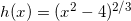Decreasing: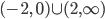| Increasing: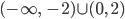Increasing:| Decreasing:both a and bnone of the aboveFind the intervals in which the following function is increasing/decreasing.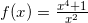Increasing: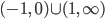| Decreasing: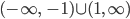Decreasing:| Increasing:both a and bnone of the aboveWhat does it mean for a function to be concave up or concave down?If part of a graph of a curve bends up, then it is said to be concave up.If part of a graph of a curve bends down, then it is said to be concave down.both a and bnone of the aboveWhat does it mean for a function to have inflection points?Inflection points occur when a derivative is set equal to zero.Inflection points occur when a second derivative is set equal to zero.Inflection points occur when concavity changes from positive to negative or vice versa.all of the abovenone of the aboveWhat interval of the following function describes its concavity?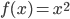Concave down:Concave up:no concavityall of the abovenone of the aboveReviewPre-calculusExtremaMinimaMaximaAbsolute and Relative Extrema (video)Interval NotationExtrema (video)/Critical Numbers ((checkpoints)How can extrema be defined for a function?How can critical numbers be calculated using derivatives?How are critical numbers related to extrema?How does Extreme Value Theorem work?Increasing/Decreasing Functions (video) (checkpoints)How can derivatives be used to find intervals of increasing and decreasing?How can derivatives be used to find relative extrema?Rolle's Theorem (video)/Mean Value Theorem (video) (Desmos Demonstration) (checkpoints)LessonConcavity and Inflection PointsHow are derivative related to intervals of concave upwards and downwards? Exit Ticket Posted on the board at the end of the block.HomeworkStudy! Help Request List Standard(s) APC.7Analyze the derivative of a function as a function in itself.Includes:comparing corresponding characteristics of the graphs of f, f', and f''​defining the relationship between the increasing and decreasing behavior of f and the sign of f'​translating verbal descriptions into equations involving derivatives and vice versaanalyzing the geometric consequences of the Mean Value Theorem;defining the relationship between the concavity of f and the sign of f"; and ​identifying points of inflection as places where concavity changes and finding points of inflection.APC.8Apply the derivative to solve problems.Includes:​analysis of curves and the ideas of concavity and monotonicityoptimization involving global and local extrema;modeling of rates of change and related rates;use of implicit differentiation to find the derivative of an inverse function;interpretation of the derivative as a rate of change in applied contexts, including velocity, speed, and acceleration; anddifferentiation of nonlogarithmic functions, using the technique of logarithmic differentiation.**AP Calculus BC will also apply the derivative to solve problems.Includes:​analysis of planar curves given in parametric form, polar form, and vector form, including velocity and acceleration vectors;​numerical solution of differential equations, using Euler’s method;​l’Hopital’s Rule to test the convergence of improper integrals and series; and​geometric interpretation of differential equations via slope fields and the relationship between slope fields and the solution curves for the differential equations.